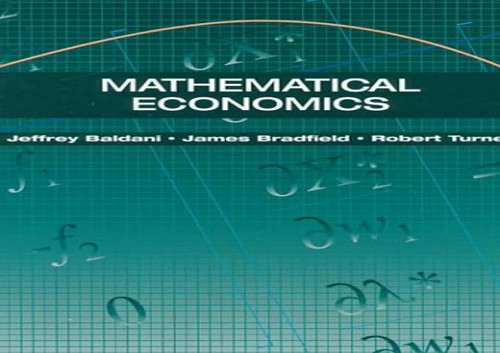• Free Shipping on all orders in Australia
• Over 7 million books in stock
• Proud to be B-Corp
• We aim to be carbon neutral by 2022
• Over 120,000 Trustpilot reviews
Item 1 of 0Mathematical Economics by Jeffrey Baldani

Condition - Very Good
\$36.99
Only 1 left

Summary

Emphasising economic applications of mathematics, this text is suitable for courses in mathematical economics at the undergraduate level. Chapters are organised in pairs so that a chapter on economic applications follows a chapter on mathematical theory.

Mathematical Economics Summary

Mathematical Economics by Jeffrey Baldani

Emphasising interesting and challenging economic applications of mathematics, this text is suitable for courses in mathematical economics at the undergraduate level. Chapters are organised in pairs so that a chapter on economic applications follows a chapter on mathematical theory. Macro applications include ISLM and the Keynesian model, and Micro applications include the Cobb-Douglas model, and utility and profit maximisation. Also covered are the economic applications of static and dynamic game theory. Features: * Chapters are organized in pairs where a chapter on mathematical theory is followed by a chapter on economic applications. This approach allows for flexibility so instructors can tailor the course to their needs. * Macroeconomic applications include ISLM and the Keynesian model, and microeconomic applications include the Cobb-Douglas model, and utility and profit maximization. * Each chapter (except Chapter 1) includes extensive end-of-chapter problems. The solutions to these problems are provided in the Instructor's Manual. * An appendix reviews basic calculus to refresh students' memories. * Economic applications of static and dynamic game theory are covered. * An extensive treatment of constrained optimization includes discussion of inequality constraints and duality.

Introduction to Mathematical Economics. Introduction to Mathematical Economic Applications. Matrix Theory. Applications of Matrix Theory to Linear Models. Multivariate Calculus: Theory. Multivariate Calculus: Applications. Multivariable Optimization Without Constraints: Theory. Multivariable Optimization Without Constraints: Applications. Constrained Optimization: Theory. Constrained Optimization: Applications. Optimization with Inequality Constraints: Theory. Optimization with Inequality Constraints. Value Functions and the Envelope Theorem: Theory. Value Functions and the Envelope Theorem: Duality and Other Applications. Static Games with Complete Information: Theory. Static Games with Complete Information: Applications. Dynamic Games with Complete Information: Theory. Dynamic Games with Complete Information: Applications.

GOR006110831
9780030981456
003098145X
Mathematical Economics by Jeffrey Baldani
Used - Very Good
Hardback
Cengage Learning, Inc
1995-10-15
500
N/A
Book picture is for illustrative purposes only, actual binding, cover or edition may vary.
This is a used book - there is no escaping the fact it has been read by someone else and it will show signs of wear and previous use. Overall we expect it to be in very good condition, but if you are not entirely satisfied please get in touch with us